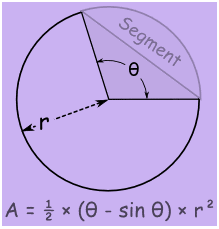# Area of a Segment of a Circle Formula

A circular segment is a region of a circle which is created by breaking apart from the rest of the circle through a secant or a chord. In other words, it is two equal halves that are divided by the circle’s arc and connected through chord by the endpoints of the arc. The formulas to calculate the area of a segment of a circle is given below. According to the definition, the part of the circular region which is enclosed between a chord and corresponding arc is known as the segment of a circle. The segment portraying a larger area is known as the major segment and the segment having a smaller area is known as a minor segment.Formula To Calculate Area of a Segment of a Circle
Area of a Segment in Radians A = (1⁄2) × r2 (θ – Sin θ)
Area of a Segment in Degrees A = (½) × r 2 × [(π/180) θ – sin θ]

### Solved Example Question

Question 1: Find the area of a segment of a circle with a central angle of 75 degrees and a radius of 5 inches.

Solution:

Given,

θ = 75

radius = r = 5 inches

Arearadians = 12 r2 (θ – sin θ)

= 12 × 52 × (75 – sin75)

= 12 × 25 × 75 –(− 0.3877)

= 12 × 25 × (75 + 0.3877)

= 12 × 25 × (75.3877)

= 12 × 1884.6925

= 942.34